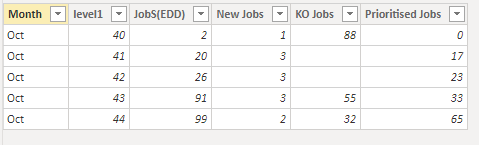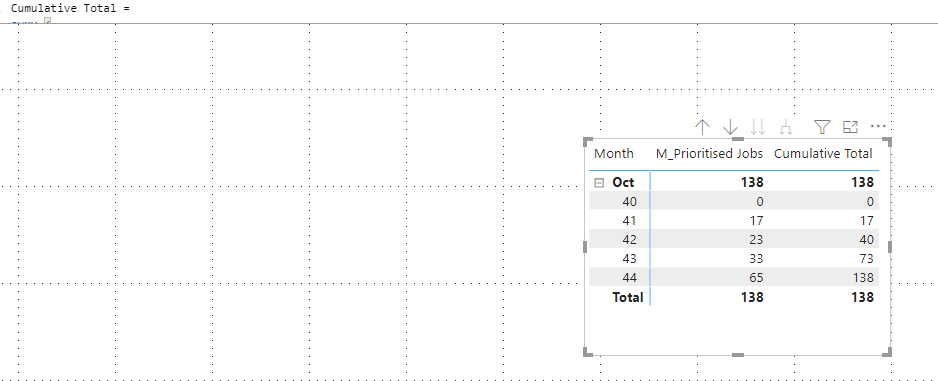cancel
Showing results for
Did you mean:Helper I

Hola, estoy intentando crear una columna con totales acumulados para la columna 'Prioritised Jobs' por semana. Esta es mi tabla actual. (40, 41, 42, etc son semanas)1 ACCEPTED SOLUTIONCommunity Support

Try sumx funtcion to calculate the sum of measure.

Here is my sample.Measure:

``M_Prioritised Jobs = SUM('Table'[Prioritised Jobs])``
``````Cumulative Total =
SUMX (
FILTER (
ALL ( 'Table' ),
'Table'[Month] = MAX ( 'Table'[Month] )
&& 'Table'[level1] <= MAX ( 'Table'[level1] )
),
[M_Prioritised Jobs]
)``````

Result is as below.Best Regards,
Rico Zhou

If this post helps, then please consider Accept it as the solution to help the other members find it more quickly.

3 REPLIES 3Helper I

Thanks, but this is not working because all the columns are measuremetsCommunity Support

Try sumx funtcion to calculate the sum of measure.

Here is my sample.Measure:

``M_Prioritised Jobs = SUM('Table'[Prioritised Jobs])``
``````Cumulative Total =
SUMX (
FILTER (
ALL ( 'Table' ),
'Table'[Month] = MAX ( 'Table'[Month] )
&& 'Table'[level1] <= MAX ( 'Table'[level1] )
),
[M_Prioritised Jobs]
)``````

Result is as below.Best Regards,
Rico Zhou

If this post helps, then please consider Accept it as the solution to help the other members find it more quickly.Super User

Column = calculate(sum([Prioritised Jobs]),filter(all(table),[Prioritised Jobs]<=earlier([Prioritised Jobs]))))

Or this, measure:

Column = calculate(sum([Prioritised Jobs]),filter(all(table),[Prioritised Jobs]<=Max([Prioritised Jobs]))))

If this post helps, please consider accepting it as the solution to help the other members find it more quickly.